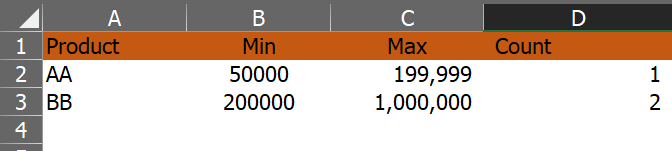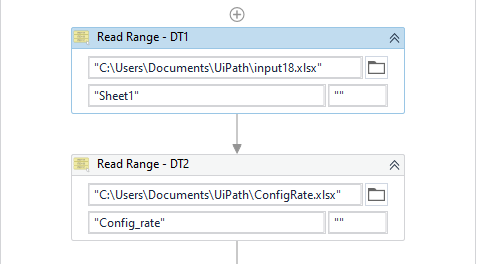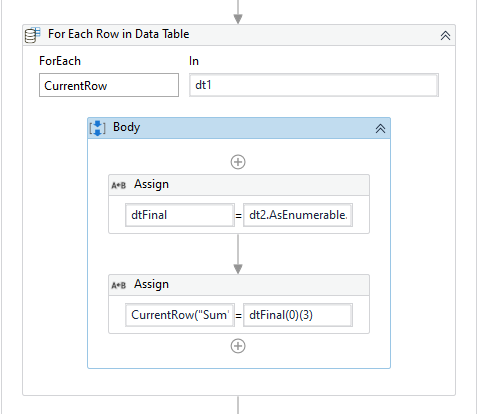# Compare Rate and write value?

I want to read Column Count in file config.And write in Column Sum as below. (compare Rate column FYP in file Config) —>In yellow highlight

ConfigRate.xlsx (19.4 KB)
input18.xlsx (10.3 KB)

@Ramya_K Can you guide me for solve it ?

1 Like

Hi @fairymemay !

To be sure to understand, you want:

• For instance for FYP (coordinate S2), it is 508,980 and because it is between 200,000 and 1,000,000 then the sum that has to be reported from column Count to column Sum is 2.
• For instance for FYP (coordinate S3), it is 149,700 and because it is between 50,000 and 199,999 then the sum that has to be reported from column Count to column Sum is 1.

Is it ?

@Hiba_B yes

Loop through each row in input file and use where query to find the sum column value.

dt_Final =
dt2.AsEnumerable.where(Function(checkRow) CInt(CurrentRow(“FYP”).ToString) >= CInt(checkRow(“Min”).ToString) And CInt(CurrentRow(“FYP”).ToString) <= CInt(checkRow(“Max”).ToString)).CopyToDataTableHope this helps.
Filter.xaml (8.1 KB)

1 Like

Hi @fairymemay !Here is another suggestion: From_count_to_sum.xaml (9.6 KB)

It uses the filter activity to do it, but basically behind the .asenumerable() that suggests @Ramya_K there is a filter in itLet us know if we missed something !

(If you see “missing activities” that means that we are not using the same Studio version. Mine in 21.10)

2 Likes

This topic was automatically closed 3 days after the last reply. New replies are no longer allowed.